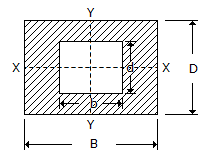Courses

# Test: Engineering Mechanics - 8

## 25 Questions MCQ Test Engineering Mechanics | Test: Engineering Mechanics - 8

Description
This mock test of Test: Engineering Mechanics - 8 for Mechanical Engineering helps you for every Mechanical Engineering entrance exam. This contains 25 Multiple Choice Questions for Mechanical Engineering Test: Engineering Mechanics - 8 (mcq) to study with solutions a complete question bank. The solved questions answers in this Test: Engineering Mechanics - 8 quiz give you a good mix of easy questions and tough questions. Mechanical Engineering students definitely take this Test: Engineering Mechanics - 8 exercise for a better result in the exam. You can find other Test: Engineering Mechanics - 8 extra questions, long questions & short questions for Mechanical Engineering on EduRev as well by searching above.
QUESTION: 1

Solution:
QUESTION: 2

Solution:
QUESTION: 3

### A body of mass m moving with a constant velocity v strikes another body of same mass m moving with same velocity but in opposite direction. The common velocity of both the bodies after collision is

Solution:
QUESTION: 4

Moment of inertia of a rectangular section having width (b) and depth (d) about an axis passing through its C.G. and parallel to the width (b), is

Solution:
QUESTION: 5

The unit of power in S.I. units is

Solution:
QUESTION: 6

According to parallel axis theorem, the moment of inertia of a section about an axis parallel to the axis through centre of gravity (i.e. IP) is given by(where, A = Area of the section, IG = Moment of inertia of the section about an axis passing through its C.G., and h = Distance between C.G. and the parallel axis.)

Solution:
QUESTION: 7

The resultant of two equal forces P making an angle θ, is given by

Solution:
QUESTION: 8

The force, by which the body is attracted, towards the centre of the earth, is called

Solution:
QUESTION: 9

Coplaner concurrent forces are those forces which

Solution:
QUESTION: 10

The power developed by a body acted upon by a torque T newton metre (N - m) and revolving at ω radian/s is given by

Solution:
QUESTION: 11

The time taken by a particle for one complete oscillation is known as periodic time.

Solution:
QUESTION: 12

The velocity ratio of a first system of pulleys with 4 pulleys is

Solution:
QUESTION: 13

One joule means that

Solution:
QUESTION: 14

The moment of inertia of a sphere of mass m and radius r, about an axis tangential to it, is

Solution:
QUESTION: 15

The velocity ratio for the first system of pulleys is(where n is the number of pulleys.)

Solution:
QUESTION: 16

The ideal angle of banking provided on the curves on roads depends upon

Solution:
QUESTION: 17

If a pendulum is taken 1 km below the earth surface in a mine, it will __________ in time.

Solution:
QUESTION: 18

The moment of inertia of a thin disc of mass m and radius r, about an axis through its centre of gravity and perpendicular to the plane of the disc is

Solution:
QUESTION: 19

The linear velocity of a body rotating at ω rad / s along a circular path of radius r is given by

Solution:
QUESTION: 20

A machine which is capable of doing work in the reversed direction, after the effort is removed, is called a non-reversible machine.

Solution:
QUESTION: 21

The forces which do not meet at one point and their lines of action do not lie on the same plane are known as

Solution:
QUESTION: 22

A non-reversible machine is also called a self-locking machine.

Solution:
QUESTION: 23

Mass moment of inertia of a thin rod about its one end is __________ the mass moment of inertia of the same rod about its mid-point

Solution:
QUESTION: 24

Moment of inertia of a hollow rectangular section as shown in the below figure about Y-Y axis, is not the same as that about X-X axis.Solution:
QUESTION: 25

The angle of the inclined plane at which a body just begins to slide down the plane, is called helix angle.

Solution: